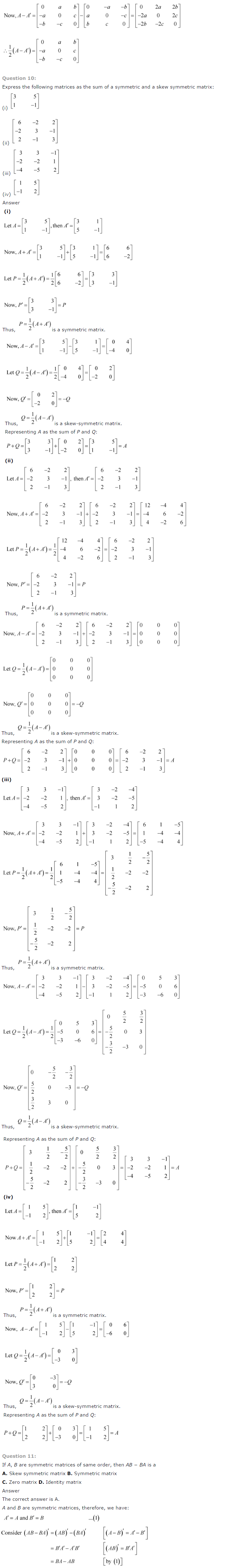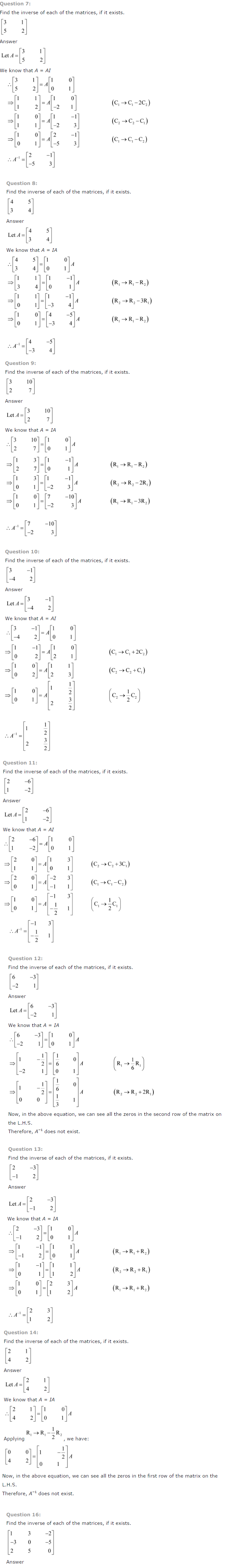The NCERT Solutions Class 12 Maths Ch 3 Matrices will help in preparation of Class 12 board exams, achieving rank in JEE (Main and Advanced) and scoring marks in other exams. The NCERT Solutions Class 12 Maths Ch 3 Matrices has Ex 3.1 Introduction, Ex 3.2 Matrix, Ex 3.3 Types of Matrices, Ex 3.4 Operations on Matrices, Ex 3.5 Transpose of a Matrix, Ex 3.6 Symmetric and Skew Symmetric Matrices, Ex 3.7 Elementary Operation (Transformation) of a Matrix, Ex 3.8 Invertible Matrices.

#### Topics and Exercises in NCERT Solution Class 12 Maths Chapter 3 Matrices:

• Ch 3 Matrices
• Ex 3.1 Introduction
• Ex 3.2 Matrix
• Ex 3.3 Types of Matrices
• Ex 3.4 Operations on Matrices
• Ex 3.5 Transpose of a Matrix
• Ex 3.6 Symmetric and Skew Symmetric Matrices
• Ex 3.7 Elementary Operation (Transformation) of a Matrix
• Ex 3.8 Invertible Matrices